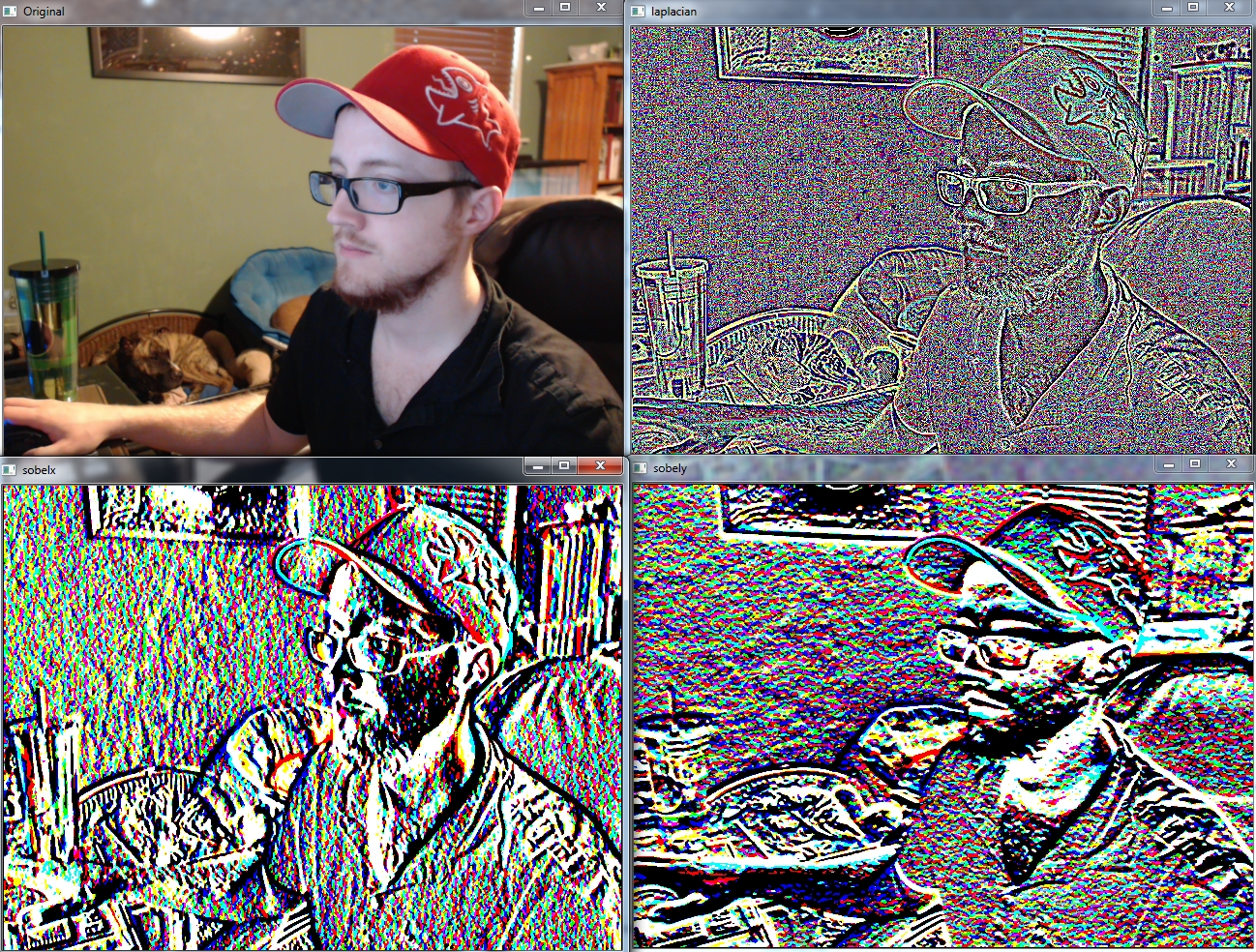## Canny Edge Detection and Gradients OpenCV Python Tutorial

Welcome to another OpenCV with Python tutorial. In this tutorial, we'll be covering image gradients and edge detection. Image gradients can be used to measure directional intensity, and edge detection does exactly what it sounds like: it finds edges! Bet you didn't see that one coming.

First, let's show some gradient examples:

```import cv2
import numpy as np

cap = cv2.VideoCapture(1)

while(1):

# Take each frame
hsv = cv2.cvtColor(frame, cv2.COLOR_BGR2HSV)

lower_red = np.array([30,150,50])
upper_red = np.array([255,255,180])

laplacian = cv2.Laplacian(frame,cv2.CV_64F)
sobelx = cv2.Sobel(frame,cv2.CV_64F,1,0,ksize=5)
sobely = cv2.Sobel(frame,cv2.CV_64F,0,1,ksize=5)

cv2.imshow('Original',frame)
cv2.imshow('laplacian',laplacian)
cv2.imshow('sobelx',sobelx)
cv2.imshow('sobely',sobely)

k = cv2.waitKey(5) & 0xFF
if k == 27:
break

cv2.destroyAllWindows()
cap.release()
```

Result:If you're wondering what the `cv2.CV_64F` is, that's the data type. ksize is the kernel size. We use 5, so 5x5 regions are consulted.

While we can use these gradients to convert to pure edges, we can also use Canny Edge detection!

```import cv2
import numpy as np

cap = cv2.VideoCapture(0)

while(1):

hsv = cv2.cvtColor(frame, cv2.COLOR_BGR2HSV)

lower_red = np.array([30,150,50])
upper_red = np.array([255,255,180])

cv2.imshow('Original',frame)
edges = cv2.Canny(frame,100,200)
cv2.imshow('Edges',edges)

k = cv2.waitKey(5) & 0xFF
if k == 27:
break

cv2.destroyAllWindows()
cap.release()```

Result:That's pretty darn good! It's not all perfect, however. Notice shadows are causing edges to be detected. One of the most obvious would be from the shadow that the blue dog bed is giving off.

In the next OpenCV tutorial, we're going to be talking about how we can search for and find identical templates of images in other images.

The next tutorial:• Drawing and Writing on Image OpenCV Python Tutorial

• Image Operations OpenCV Python Tutorial

• Image arithmetics and Logic OpenCV Python Tutorial

• Thresholding OpenCV Python Tutorial

• Color Filtering OpenCV Python Tutorial

• Blurring and Smoothing OpenCV Python Tutorial

• Morphological Transformations OpenCV Python Tutorial

• Canny Edge Detection and Gradients OpenCV Python Tutorial
• Template Matching OpenCV Python Tutorial

• GrabCut Foreground Extraction OpenCV Python Tutorial

• Corner Detection OpenCV Python Tutorial

• Feature Matching (Homography) Brute Force OpenCV Python Tutorial

• MOG Background Reduction OpenCV Python Tutorial

• Haar Cascade Object Detection Face & Eye OpenCV Python Tutorial